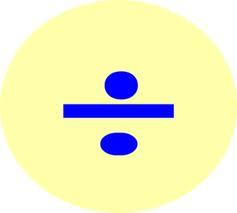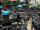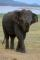# Guess number

With what number should be divided number 9727 to be reduced by 70%?

Result

x =  3.3

#### Solution:

$x = \dfrac{ 100}{ 100 - 70 } = 3.3$Our examples were largely sent or created by pupils and students themselves. Therefore, we would be pleased if you could send us any errors you found, spelling mistakes, or rephasing the example. Thank you!

Leave us a comment of this math problem and its solution (i.e. if it is still somewhat unclear...):Be the first to comment!Tips to related online calculators
Do you have a linear equation or system of equations and looking for its solution? Or do you have quadratic equation?

#### You need to know the following knowledge to solve this word math problem:

We encourage you to watch this tutorial video on this math problem:

## Next similar math problems:

1. Dropped sheetsThree consecutive sheets dropped from the book. The sum of the numbers on the pages of the dropped sheets is 273. What number has the last page of the dropped sheets?
2. Unknown number 11That number increased by three equals three times itself?
3. Find xSolve: if 2(x-1)=14, then x= (solve an equation with one unknown)
4. Volatile percentsKent is working as a forex trader. Suffered a loss of 65.8 percent. Calculate what percentage must now earn to lift from loss?
5. ClassIn 7.C clss are 10 girls and 20 boys. Yesterday was missing 20% of girls and 50% boys. What percentage of students missing?
6. Negative in equation2x + 3 + 7x = – 24, what is the value of x?
7. Simple equation 1035= 7*3*x what is x?
8. 100 %How much is 100%, if 17 % is 1169?
9. Seeds 2How many seeds germinated from 1000 pcs, when 23% no emergence?
10. IronIron ore contains 57% iron. How much ore is needed to produce 20 tons of iron?
11. NormThree workers planted 3555 seedlings of tomatoes in one dey. First worked at the standard norm, the second planted 120 seedlings more and the third 135 seedlings more than the first worker. How many seedlings were standard norm?
12. Simple equation 9Solve the following equation: -8y+5=-9y+9
13. Simple equationSolve for x: 3(x + 2) = x - 18
14. Forest nurseryIn the forest nursery after winter, they found that 1/10 stems died out of them. For them, they land 193 new spruces. How many spruces are in the forest nursery?
15. Equation 29Solve next equation: 2 ( 2x + 3 ) = 8 ( 1 - x) -5 ( x -2 )
16. Simple equation 8Solve the following equation: 36=-(1+7x)-6(-7-x)
17. Simple equationSolve the following simple equation: 2. (4x + 3) = 2-5. (1-x)